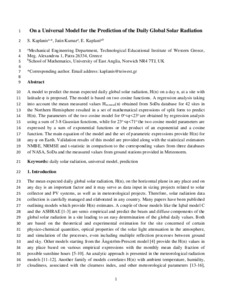# On a universal model for the prediction of the daily global solar radiation

Kaplanis, Socrates, Kumar, Jatin and (2016) On a universal model for the prediction of the daily global solar radiation. Renewable Energy, 91. pp. 178-188. ISSN 0960-1481Preview PDF (manuscript_SKaplanis_etal_UniversalModel) - Accepted Version Available under License Creative Commons Attribution Non-commercial No Derivatives. Download (1MB) | Preview

## Abstract

A model to predict the mean expected daily global solar radiation, H(n) on a day n, at a site with latitude φ is proposed. The model is based on two cosine functions. A regression analysis taking into account the mean measured values Hm.meas(n) obtained from SoDa database for 42 sites in the Northern Hemisphere resulted in a set of mathematical expressions of split form to predict H(n). The parameters of the two cosine model for 0o<φ<23o are obtained by regression analysis using a sum of 3-8 Gaussian functions, while for 23o<φ<71o the two cosine model parameters are expressed by a sum of exponential functions or the product of an exponential and a cosine function. The main equation of the model and the set of parametric expressions provide H(n) for any φ on Earth. Validation results of this model are provided along with the statistical estimators NMBE, NRMSE and t-statistic in comparison to the corresponding values from three databases of NASA, SoDa and the measured values from ground stations provided in Meteonorm.

Item Type: Article daily solar radiation,universal model,prediction Faculty of Science > School of Mathematics Pure Connector 26 Jan 2016 13:00 22 Oct 2022 00:43 https://ueaeprints.uea.ac.uk/id/eprint/56766 10.1016/j.renene.2016.01.037View Item